Courses
Courses for Kids
Free study material
Offline Centres
More

# Current Electricity Chapter - Physics JEE MainLast updated date: 01st Dec 2023
Total views: 21k
Views today: 1.21k## Concepts of Current Electricity JEE Main Physics

Current Electricity is a crucial chapter in the JEE Main syllabus, and it's all about understanding how electric currents work and how they interact with materials. In simple terms, it's like learning how electricity flows in wires and devices. This chapter is fundamental as it forms the basis for a multitude of advanced electrical and electronic concepts.

In this article, explore various aspects of Current Electricity, including key concepts like Electric Current (the flow of electricity), Ohm's Law (which explains the relationship between voltage and current), Electrical Resistance (how materials slow down electric flow), and Drift Velocity (how fast electrons move). We will also discuss different materials' resistances, Ohmic and non-Ohmic conductors, and the basics of Electrical Energy and Power. Understanding electrical resistivity, the color code for resistors, and how resistors can be combined in series and parallel is also on our list. Plus, we will delve into the temperature effects on resistance.

## JEE Main Physics Chapters 2024

### Important Topics of Current Electricity Chapter

• Electric current density and drift velocity

• Ohm’s law and temperature depend on resistivity.

• Grouping of Resistors

• Colour code of carbon resistor

• Grouping of cells

• Kirchhoff’s Law

• Wheatstone bridge

• Metre bridge

• Potentiometer

### JEE Main Current Electricity Solved Examples

1. The internal resistance of a cell of emf 2V is 0.1 Ω. It is connected to a resistance of 3.9 Ω. Find the voltage across the cell.

Sol: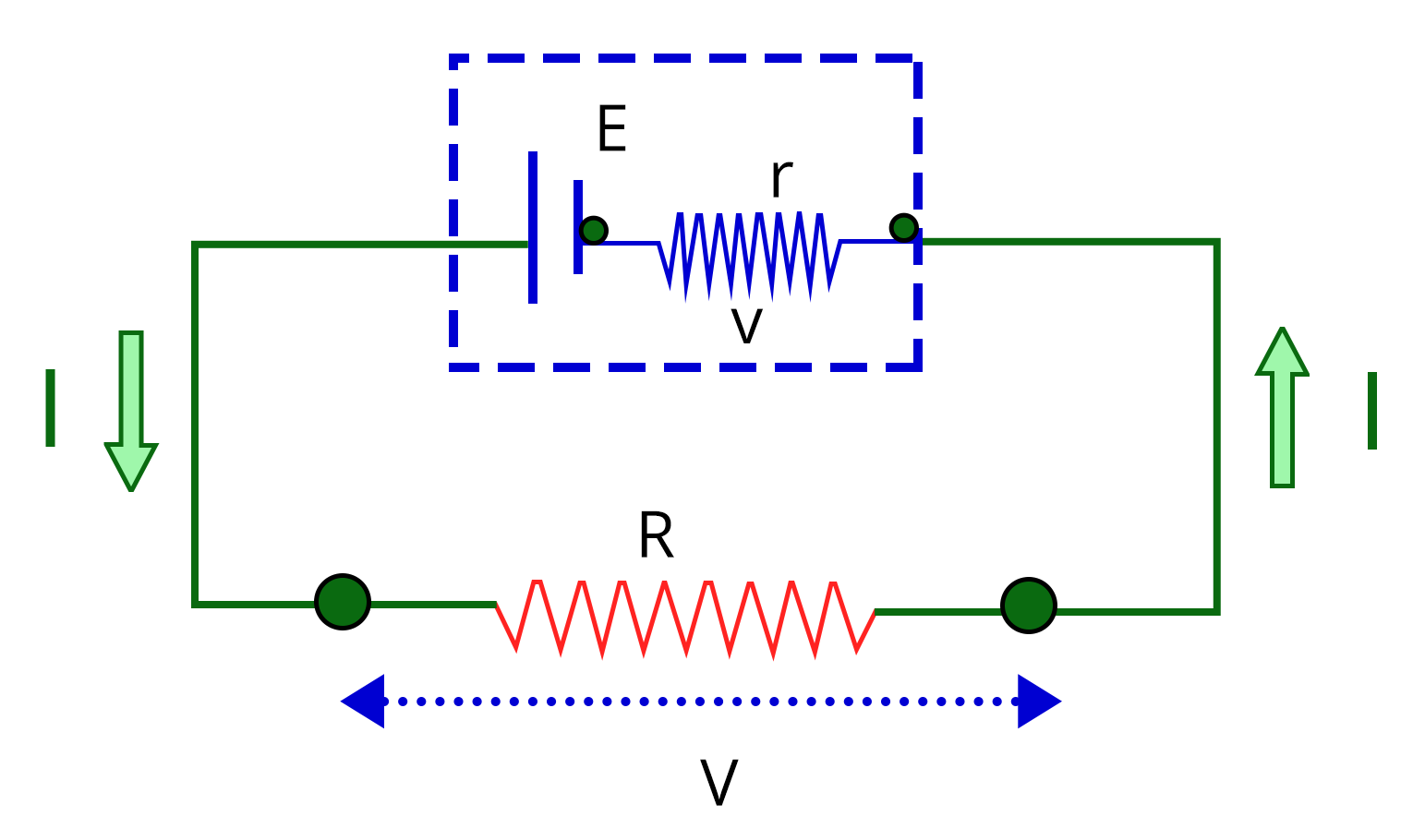Given:

Emf of the cell, E = 2V

Internal resistance of the cell, r = 0.1 Ω

Resistance connected to the cell, R = 3.9 Ω

Let V be the terminal voltage across the cell.

We know that the voltage drop across the resistor,

$V=IR$....(1)

Applying Kirchhoff's voltage law,

$E-V-Ir=0$

$E-IR-Ir=0$

$2V-3.9I-0.1I=0$

$I=\dfrac{2}{4}=0.5~A$

Substitute the value of current I in equation (1) to obtain the voltage across the cell V.

$V=0.5\times 3.9=1.95~V$

Therefore, the voltage across the cell is 1.95 V

Key point: Since resistor R and the cell are in parallel connection, the voltage across them are equal.

2. The resistance of a wire is R ohm. If it is melted and stretched to n times its original length, its new resistance will be_________.

Sol:

Given:

The resistance of the wire is R.

Let the length and area of the cross-section of wire be l and A, respectively and the resistivity of the wire be 𝜌.

If the length of the wire is stretched to n times, then the area of the cross-section of the wire is reduced by n times.

Let the length and area of the cross-section of the stretched wire be l’ and A’, respectively.

The resistance of the stretched wire is given by

$R'=\rho\dfrac{l'}{A'}$

$R'=\rho\dfrac{(nl)}{\left(\dfrac{A}{n}\right)}$

$R'=\rho\dfrac{n^2l}{A}$

$R'=n^2\times\left(\rho\dfrac{l}{A}\right)$

$R'=n^2R$

Therefore, the resistance of the stretched wire is n2 times the initial resistance.

Key point: When a wire is stretched, its length increases and the area of cross-section decreases while keeping the volume constant.

### Previous Years’ Questions from JEE Paper

1. Five equal resistances are connected in a network as shown in the figure. The net resistance between points A and B is______. (Feb -2021)

a. 3R/2

b. R/2

c. R

d. 2R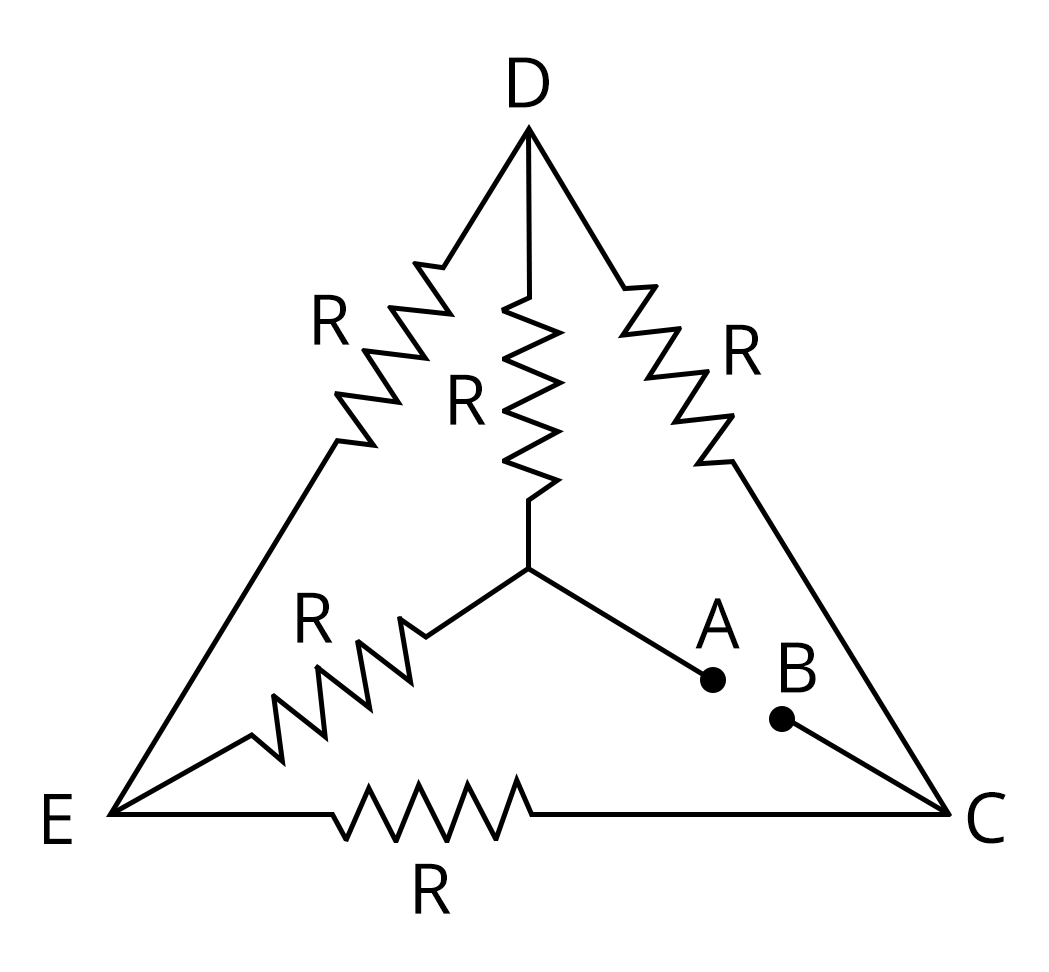Sol:

If you observe the diagram closely, you can see that the above circuit diagram is a balanced Wheatstone bridge since all the resistances are the same. Therefore, it satisfies the condition

$\dfrac{R_2}{R_{1}}=\dfrac{R_4}{R_3}$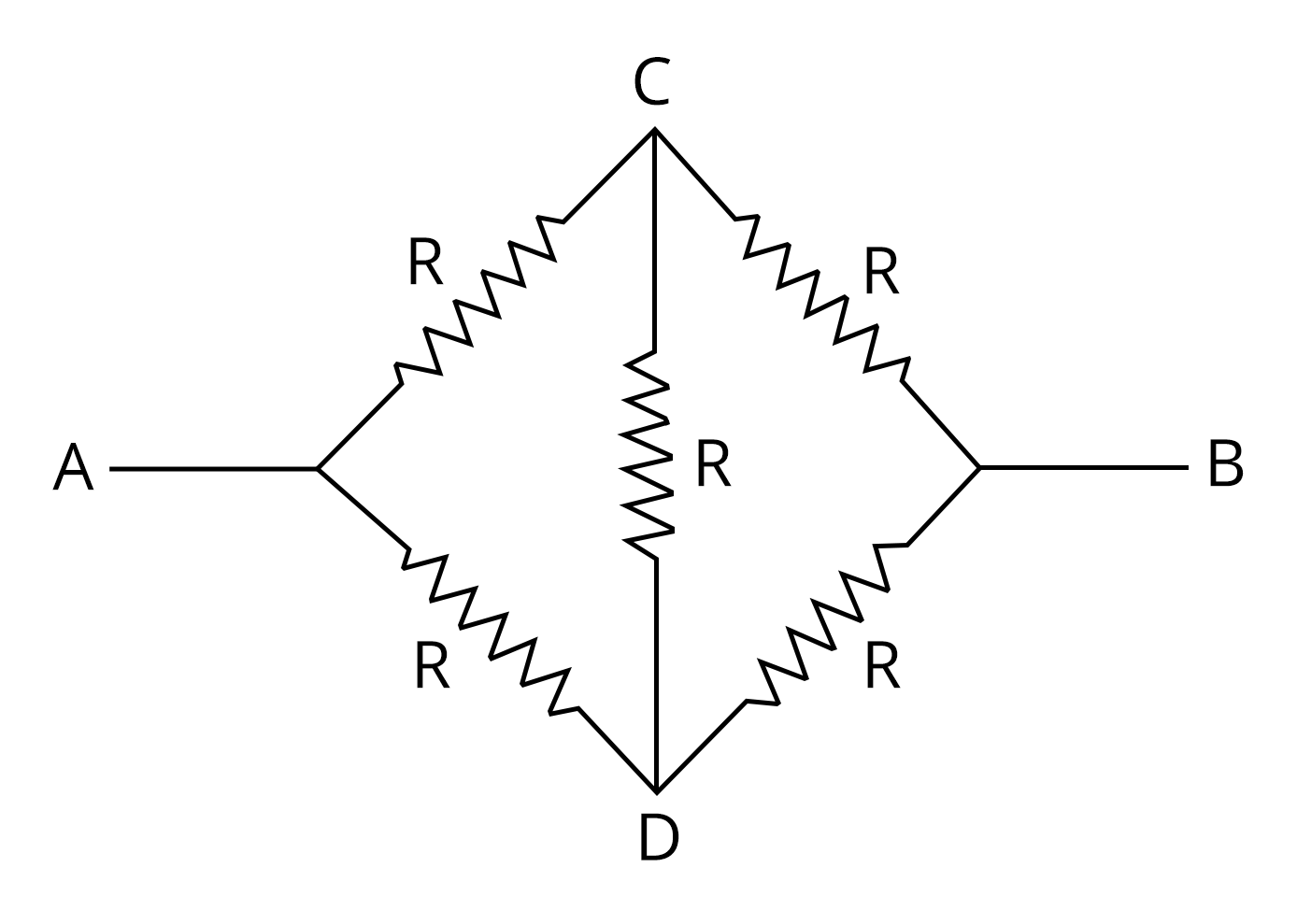Since it is balanced, the current does not pass through the resistor in the bridge and can be removed while calculating the effective resistance.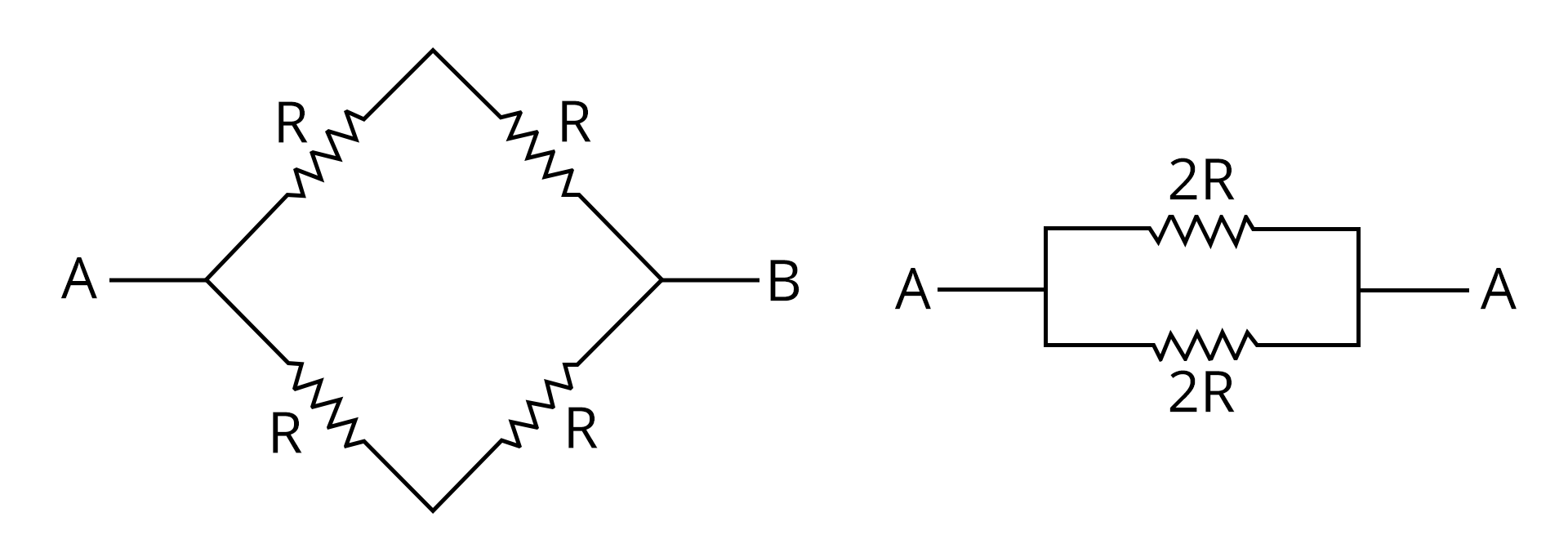The two 2R resistors are in parallel, so the equivalent resistance between A and B is

$R_{eq}=\dfrac{2R\times 2R}{2R+2R}$

$R_{eq}=\dfrac{4R^2}{4R}$

$R_{eq}=R$

Therefore, the equivalent resistance between A and B is R and the correct answer is option C.

Key point to remember: When a difficult circuit diagram having resistors are given, always look for the possibility of a balanced Wheatstone bridge.

2. The length of a potentiometer wire is 1200 𝑐𝑚 and it carries a current of 60 𝑚𝐴. For a cell of emf 5 𝑉 and internal resistance of 20Ω, the null point on it is found to be at 1000 𝑐𝑚. The resistance of the whole wire is_____. (JEE 2020)

a. 80Ω

b. 100Ω

c. 120Ω

d. 60Ω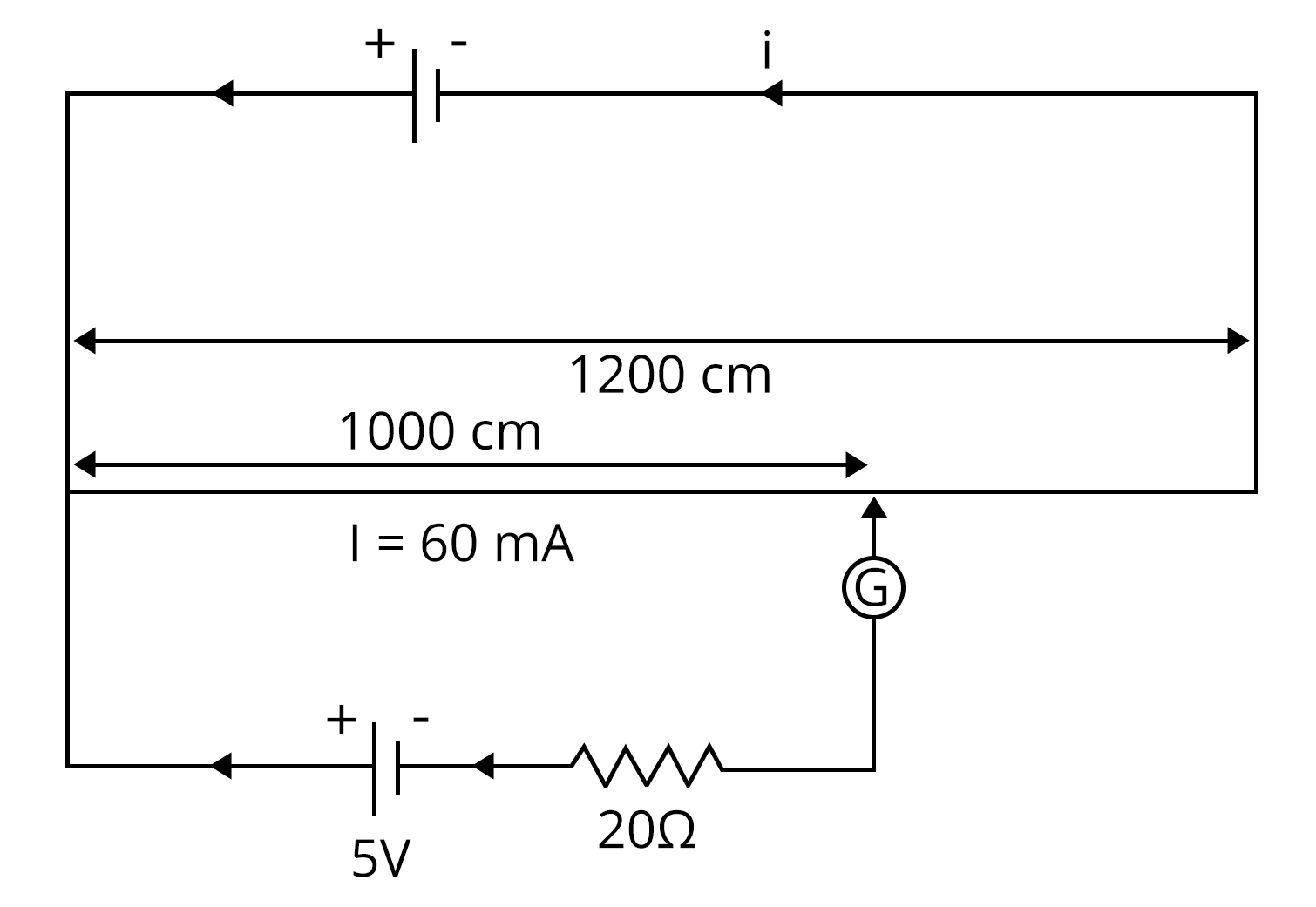Sol:

Given:

The null point of the potentiometer = 1000 cm

The current through the potentiometer wire, I = 60 mA

Emf of the cell in the secondary circuit, E = 5 V

At the null point, no current passes through the galvanometer and hence no current is drawn from the cell. Therefore, the emf of the cell is equal to the potential difference across the section of the wire having a length of 1000 cm.

So, the resistance of the wire of 1000 cm (R1) can be calculated.

$R_1=\dfrac{E}{I}$

$R_1=\dfrac{5~V}{60~\text{mA}}$

$R_1=83.33~ \Omega$

Let the resistance of the wire per unit length be r.

$r=\dfrac{83.33~ \Omega}{1000~\text{cm} }$

$r=83.33\times 10^{-3}~ \Omega/cm$

Now, the resistance of the 1200 cm potentiometer wire (R) is obtained by

$R=83.33\times 10^{-3}~ \Omega/cm~\times 1200~\text{cm}$

$R=100~\Omega$

Therefore, the correct answer is option B.

Key point to remember: At the balancing point, no current is drawn from the cell and hence the terminal voltage and emf are equal.

### Practice Questions

1. A uniform wire of resistance 9 Ω is cut into three equal parts. They are connected in the form of an equilateral  triangle ABC. A cell of emf 2V and negligible internal  resistance is connected across B and C. Potential difference across AB is____. (Ans: 1 V)

2. A filament bulb (500 W, 100V) is to be used in a 230 V main supply. When a resistance R is connected in series, it works perfectly and consumes 500 W. The value of R is_______. (Ans: 26 Ω)

## JEE Main Physics Current Electricity Study Materials

Here, you'll find a comprehensive collection of study resources for Current Electricity designed to help you excel in your JEE Main preparation. These materials cover various topics, providing you with a range of valuable content to support your studies. Simply click on the links below to access the study materials of Current Electricity and enhance your preparation for this challenging exam.

 JEE Main Current Electricity Study Materials JEE Main Current Electricity Notes JEE Main Current Electricity Important Questions JEE Main Current Electricity Practice Paper

## JEE Main Physics Study and Practice Materials

Explore an array of resources in the JEE Main Physics Study and Practice Materials section. Our practice materials offer a wide variety of questions, comprehensive solutions, and a realistic test experience to elevate your preparation for the JEE Main exam. These tools are indispensable for self-assessment, boosting confidence, and refining problem-solving abilities, guaranteeing your readiness for the test. Explore the links below to enrich your Physics preparation.

 JEE Main Physics Study and Practice Materials JEE  Main Physics Previous Year Question Papers JEE Main Physics Mock Test JEE Main Physics Formula JEE Main Sample Paper JEE Main Physics Difference Between

### Conclusion

In this guide, we'll dive into the world of Current Electricity, a key topic in JEE Main physics. We'll demystify the core concepts, providing simple explanations and problem-solving strategies. You'll learn about electric circuits, Ohm's law, and how to calculate current and voltage. We've compiled everything you need in one place, including free downloadable PDFs with detailed explanations and practice questions. This resource is a valuable tool to help you ace your exams.

## FAQs on Current Electricity Chapter - Physics JEE Main

1. What is the weightage of the current electricity in JEE?

Around 2-3 questions are asked in JEE from various parts of the chapter. Most of the questions are based on electric current example problems and questions can also be asked by linking them to concepts from other chapters.

2. What section has to be given more importance in the chapter on current electricity?

More focus must be laid on solving electric current example problems and getting the answer without committing any mistakes. Therefore, students should be able to solve any problems related to this chapter by practising more and more problems.

3. How to score good marks in JEE from the chapter on Current Electricity?

First of all, learn and understand the concepts and current electricity formulae in each chapter in depth. This should be followed by solving all the problems given in NCERT textbooks. Then go through the question papers of the last 20 years. Try to solve them and observe the pattern to score good marks in JEE.

### Repeaters Course for JEE 2022 - 23## Notice board

JEE News
JEE Blogs